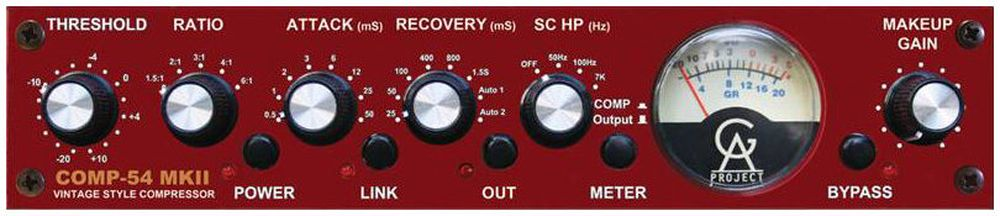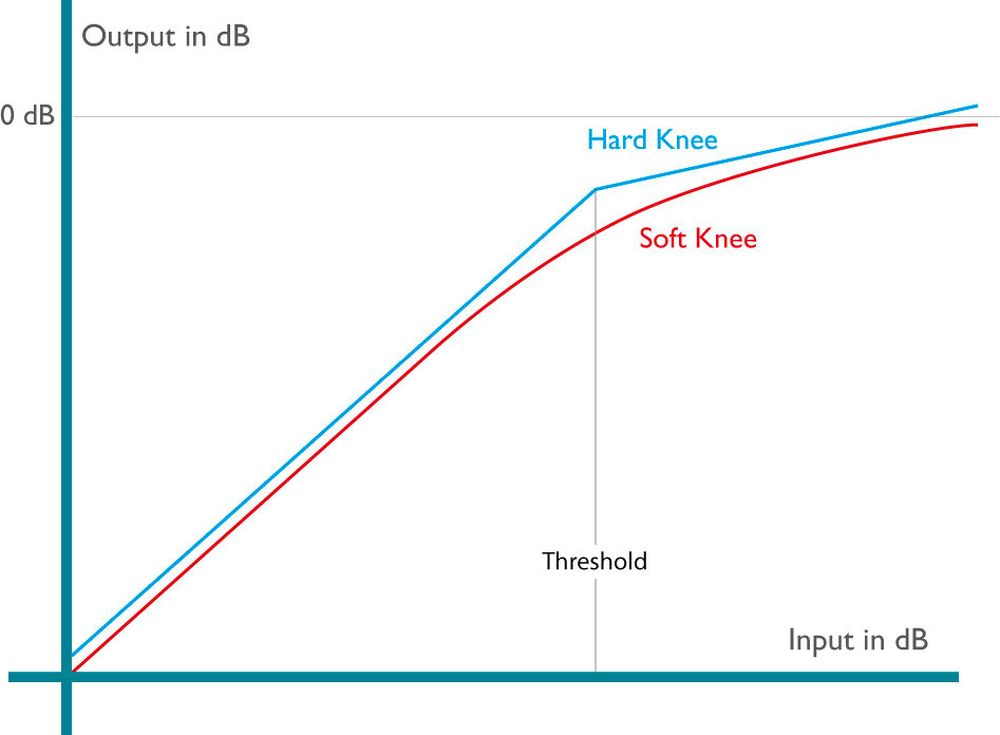## 4. Static parameters

The operating principle of a compressor is characterized by the following static parameters:

### Threshold

The threshold is the input level above which the compressor starts to attenuate the signal. Signals below the threshold pass through the compressor unprocessed. The threshold is set in dB in relation to the compressor's operating level. Therefore, it is usually a negative number (e.g. -9 dB relative to 0 dB).

### Ratio

The compression ratio is the proportion of changes of the input level to the corresponding changes of the output level. For example, a ratio of 4:1 means that an input level change of 4 dB results in an output level change of 1 dB.

### Gain reduction

The gain reduction is indicated in dB on a level meter. It is not constant, but increases progressively as the threshold is exceeded. A signal level of 4 dB above the threshold results in a different gain reduction than a level of 16 dB above the threshold. At a ratio of 4:1, the gain reduction will amount to approximately 3 dB in the first case, while it will be about 12 dB in the latter.

### Makeup gain

As a general rule, the input level is initially adjusted so that it matches the compressor's operating level (0 dB on the level meter). Because the threshold lies below the operating level (e.g. at -15 dB), a given ratio will lead to a corresponding gain reduction, attenuating the signal. This is compensated for by using the makeup gain to raise the output level. Ultimately, this means that the loudest portions of the signal (those above the threshold) are attenuated, while the level of the quieter portions is increased. The dynamic range is compressed.

The following chart illustrates the relationship between these parameters.Threshold, ratio and makeup gain are adjustable parameters of an audio compressor. Their settings determine the gain reduction, which is shown on a level meter.Golden Age Project Comp-54 MkII

As you can see in the above chart, the compression graph has a sharp kink at the threshold. The gain reduction has a linear characteristic. This means that the use of the compressor may be audibly evident, which is undesirable in many cases. For this reason, many compressors allow for a switching between so-called „hard knee“ and „soft knee“ characteristics. At the soft knee setting, the graph follows a gradual slope instead of kinking sharply.•••